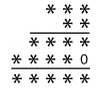# Search by Topic

#### Resources tagged with Algorithms similar to First Forward Into Logo 5: Pen Up, Pen Down:

Filter by: Content type:
Age range:
Challenge level:

##### Other tags that relate to First Forward Into Logo 5: Pen Up, Pen Down
STEM - General. Practical Activity. Mathematical modelling. Chemistry. Recursion. Programming. Real world. Sport. Logo. Algorithms.

### There are 12 results

Broad Topics > Decision Mathematics and Combinatorics > Algorithms### Tournament Scheduling

##### Age 11 to 16

Scheduling games is a little more challenging than one might desire. Here are some tournament formats that sport schedulers use.### Medal Muddle

##### Age 11 to 14 Challenge Level:

Countries from across the world competed in a sports tournament. Can you devise an efficient strategy to work out the order in which they finished?### On What Day Did it Happen?

##### Age 5 to 14

Read this article to find out the mathematical method for working out what day of the week each particular date fell on back as far as 1700.### Long Multiplication

##### Age 11 to 14 Challenge Level:

A 3 digit number is multiplied by a 2 digit number and the calculation is written out as shown with a digit in place of each of the *'s. Complete the whole multiplication sum.### Alphabet Soup

##### Age 11 to 14 Challenge Level:

This challenge is to make up YOUR OWN alphanumeric. Each letter represents a digit and where the same letter appears more than once it must represent the same digit each time.### Kids

##### Age 11 to 14 Challenge Level:

Find the numbers in this sum### Tis Unique

##### Age 11 to 14 Challenge Level:

This addition sum uses all ten digits 0, 1, 2...9 exactly once. Find the sum and show that the one you give is the only possibility.##### Age 7 to 14 Challenge Level:

Watch our videos of multiplication methods that you may not have met before. Can you make sense of them?### Skeleton

##### Age 11 to 14 Challenge Level:

Amazing as it may seem the three fives remaining in the following `skeleton' are sufficient to reconstruct the entire long division sum.### Funny Factorisation

##### Age 11 to 14 Challenge Level:

Using the digits 1 to 9, the number 4396 can be written as the product of two numbers. Can you find the factors?### X Marks the Spot

##### Age 11 to 14 Challenge Level:

When the number x 1 x x x is multiplied by 417 this gives the answer 9 x x x 0 5 7. Find the missing digits, each of which is represented by an "x" .### Slippy Numbers

##### Age 11 to 14 Challenge Level:

The number 10112359550561797752808988764044943820224719 is called a 'slippy number' because, when the last digit 9 is moved to the front, the new number produced is the slippy number multiplied by 9.